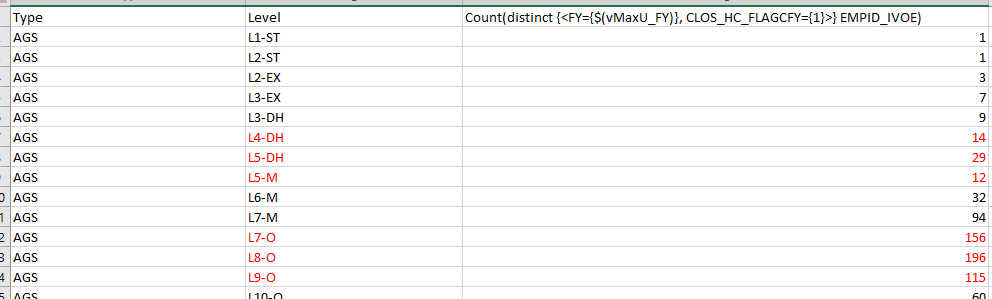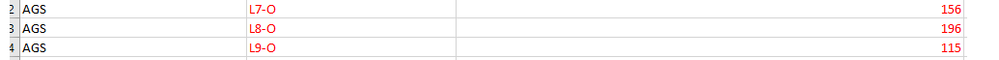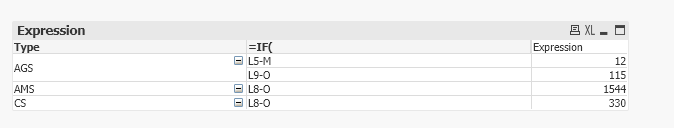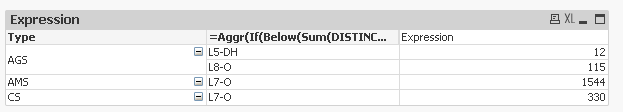# QlikView App Dev

Discussion Board for collaboration related to QlikView App Development.

Announcements
Join this live chat April 6, 10AM EST - QlikView to Qlik Sense REGISTER
cancel
Showing results for
Search instead for
Did you mean:Specialist

## How to write expression for below requirement

Hello All,

Need  help in achieving a  requirement , Having a sample data in below format ,With two Dimensions(Type,Level) and a Expression

The requirement :

Every Type has L1 to L10 values , so the requirement is instead of showing all the level for each Type, show only those level which satisfy  below condition

Condition :   N+1/N > 1   and N+2/N+1<1

For ExampleNOTE:Every row value is considered as N and top of that value is considered as N+1,N+2

From the above pic  N=L9-O (115) and N+1=L8-O(196) AND N+2=L7-O(156)

so by the condition  =     N+1/N > 1   and N+2/N+1<1

196/115  >1 true

and

156/196  <1   true

so the  condition is satisfied ,  so   for Type AGS one level will be   L9-O  (N)

so i have achieved this requirement by writing below expression in Dimension

IF((
Aggr(below(Count(distinct {<FY={\$(vMaxU_FY)}, CLOS_HC_FLAGCFY={1}>} EMPID_IVOE))
, Type
, (LevelNo,(NUMERIC, DESCENDING))
)
/
Aggr(Count(distinct {<FY={\$(vMaxU_FY)}, CLOS_HC_FLAGCFY={1}>} EMPID_IVOE), Type, Level)
) > 1

and

(
Aggr(below(Count(distinct {<FY={\$(vMaxU_FY)}, CLOS_HC_FLAGCFY={1}>} EMPID_IVOE), 2)
, Type
, (LevelNo,(NUMERIC, DESCENDING))
)
/
Aggr(below(Count(distinct {<FY={\$(vMaxU_FY)}, CLOS_HC_FLAGCFY={1}>} EMPID_IVOE))
, Type
, (LevelNo,(NUMERIC, DESCENDING))
)
) < 1
,
(Level)
)

By above expression i am getting N value from Level ,but want i need is instead of N value show N+1 value

in the above case instead of  L9-O i need to show L8-O   ,if N value is satisfied the condition then pick N+1 has out put,

so in the above expression In the place of LEVEL. wat should i need to write

Thanks

1 Solution

Accepted SolutionsMVP

@kunkumnaveen wrote:For AGS instead of showing  L5-M,L9-O  (N)as Dimension values  i need to show L5-DH and L8-O   (N+1)

How about the Level for AMS and CS? Would they also change to L7-O?Calculated dimension

```=Aggr(  If(    Below(Sum(DISTINCT {<FY={'2018'}>} Expression))/    Sum(DISTINCT {<FY={'2018'}>} Expression) > 1    and
Below(Sum(DISTINCT {<FY={'2018'}>} Expression), 2)/    Below(Sum(DISTINCT {<FY={'2018'}>} Expression)) < 1  , Below(Level)), Type, (LevelNo,(NUMERIC, DESCENDING)))```

The only thing I really changed was to use Below(Level) instead of Level. But I also removed the multiple usage of Aggr() as it seems that you were essentially aggregating on the same fields. If Level and levelNo are not one to one for each Type, then you might have to change back to your original calculated dimension and use Below(Level).

7 RepliesMVP

Would you be able to share a qvw sample to see what you already have and work with it to get you to what you want?Specialist
Author

Hello  Sunny,

As requested i am attaching a sample QVW file ,with partial achieved calculated Dimension expressionRequirement

For AGS instead of showing  L5-M,L9-O  (N)as Dimension values  i need to show L5-DH and L8-O   (N+1)Specialist
AuthorSpecialist
Author

Hello any suggestion ,on above requirementMVP

Checking it nowMVP

@kunkumnaveen wrote:For AGS instead of showing  L5-M,L9-O  (N)as Dimension values  i need to show L5-DH and L8-O   (N+1)

How about the Level for AMS and CS? Would they also change to L7-O?Calculated dimension

```=Aggr(  If(    Below(Sum(DISTINCT {<FY={'2018'}>} Expression))/    Sum(DISTINCT {<FY={'2018'}>} Expression) > 1    and
Below(Sum(DISTINCT {<FY={'2018'}>} Expression), 2)/    Below(Sum(DISTINCT {<FY={'2018'}>} Expression)) < 1  , Below(Level)), Type, (LevelNo,(NUMERIC, DESCENDING)))```

The only thing I really changed was to use Below(Level) instead of Level. But I also removed the multiple usage of Aggr() as it seems that you were essentially aggregating on the same fields. If Level and levelNo are not one to one for each Type, then you might have to change back to your original calculated dimension and use Below(Level).Specialist
Author

Thanks You,It worked